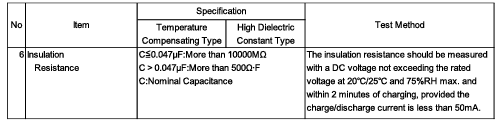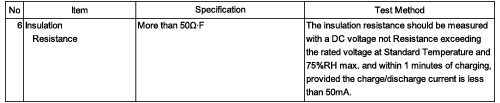# Ceramic Capacitors FAQ

Characteristics

What are the leakage current specification values for DC?

Leakage current specification values are not prescribed for DC, and are instead specified by the insulation resistance value.
The leakage current can be estimated as follows from the insulation resistance specification value and the rated voltage of the item using the formula I = V/R.
However, note that this is merely the value calculated based on the insulation resistance specification value specified by Murata, and only the insulation resistance item is guaranteed.
1. Method of obtaining the leakage current from the insulation resistance specification value
Example: GRM155B31H103KA88
(1) Check the insulation resistance specification value (guaranteed performance) of the product name GRM155B31H103KA88.(2) The GRM155B31H103KA88 capacitance is less than 0.047μF, so the insulation resistance specification value is 10,000 MΩ or more.
(3) Substitute the insulation resistance specification value of 10,000 MΩ and the rated voltage of 50 V for the product name GRM155B31H103KA88 into the formula I = V/R.
(4) I = 50/10,000 M
(5) I (leakage current) = 0.005 μA or less
2. Method of obtaining the insulation resistance specification value from ΩF and calculating the leakage current
Example: GRM188B30J106ME47
(1) Check the insulation resistance specification value (guaranteed performance) of the product name GRM188B30J106ME47.(2) According to the table, the insulation resistance specification value of the GRM188B30J106ME47 is 50 ΩF or more.
(3) The ΩF unit indicates that the value is the product of the resistance and the capacitance, so the insulation resistance is obtained by dividing 50 ΩF by the capacitance value for that product number.
(4) Insulation resistance = 50 ΩF/10 μF
(5) Insulation resistance = 5 MΩ (μ = 10-6, M = 106)
(6) Substitute the insulation resistance specification value of 5 MΩ and the rated voltage of 6.3 V for the product name GRM188B30J106ME47 into the formula I = V/R.
(7) I = 6.3/5 M
(8) I (leakage current) = 1.26 μA or less
*What is ΩF?
Ohm Farads (ΩF) is one of the units used to represent insulation resistance.
When the insulation resistance specification value is specified as the product of the nominal capacitance value and the insulation resistance (CR product), it is expressed in ΩF units.
Generally speaking, the insulation resistance is determined per unit capacitance (μF). However, in case of high-capacitance capacitors where the insulation resistance varies according to the capacitance, ΩF is used as the unit and the insulation resistance specification value is determined according to the capacitance value.

We appreciate your cooperation with the FAQ improvement questionnaire.